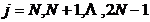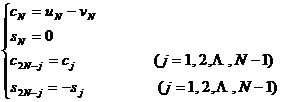§5   Fast Fourier Transform

1.         Finite discrete Fourier transform

[ Various Forms of Finite Discrete Fourier Transform ]

 real (or complex) sequence f ( kh ) Finite discrete Fourier transform and its inversion formula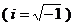hd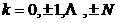( N is a positive integer)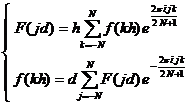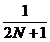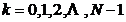( N is a positive integer)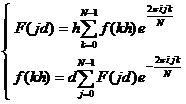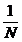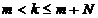( k , N is an integer)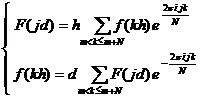[ Convolution and its properties ]   Let the real (or complex) sequence g ( kh ) be a sequence with period Nh , which is called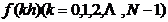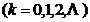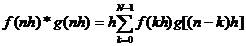is the convolution of the sequences f and g . Let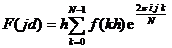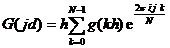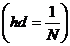then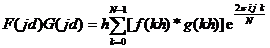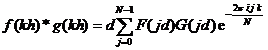Second, the     fast Fourier transform algorithm

The Fast Fourier Transform ( FFT ) algorithm is a fast method for computing finite discrete Fourier transforms .

[ FFT algorithm of complex sequence ] To calculate the finite discrete Fourier transform of the complex sequence { z k } , is to calculate the form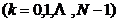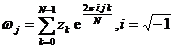,The finite term sum of . For the inversion formula, the calculation method is similar .

Let N = 2 m , ,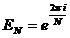then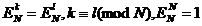set again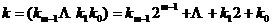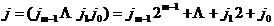are the binary representations of k and j , respectively , and take the value 0 or 1. Then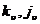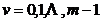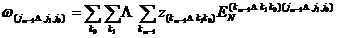because =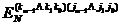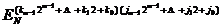=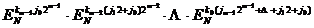=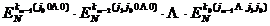so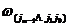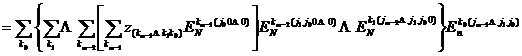This leads to the recursive formula: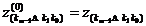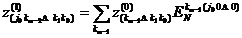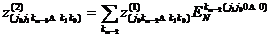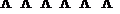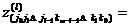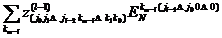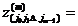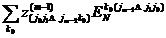Finally there is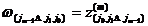[ FFT Algorithm for Real Sequences ] Finite Discrete Fourier Cosine Transforms and Sine Transforms to be Calculated for Real Sequences with 2 N ( N = 2 m ) Elements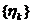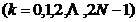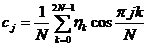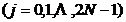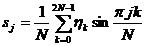The FFT algorithm can be used first for complex sequences

z k =x k +iy k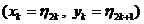calculate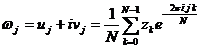And c j , s j use the following formula to find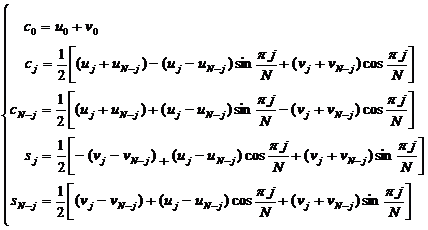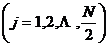As for c j , the value of s j is when